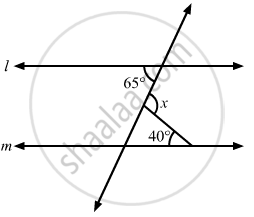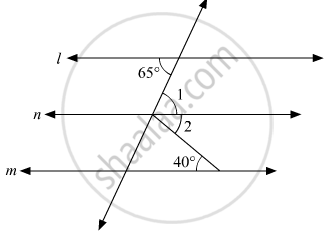# In the Given Figure, If L || M, Then X = - Mathematics

MCQ

In the given figure, if l || m, then x =• 105°

• 65°

• 40°

• 25°

#### Solution

The given figure:Let us draw a line n parallel to l and m.

Thus, we can say that  l || m ||n.

Also, from the figure we get :

x = ∠1 + ∠2                ...(i)

Since. l || n

Thus, alternate interior opposite angles are equal. That is,

∠1 = 65°                    ...(ii)

Since m||n.

Thus, alternate interior opposite angles are equal. That is,

∠2 = 40°                 ...(iii)

On substituting, equation (ii) and (iii) in (i):

x = 40°+65°

x = 105°

Concept: Parallel Lines and a Transversal
Is there an error in this question or solution?
Chapter 10: Lines and Angles - Exercise 10.6 [Page 56]

#### APPEARS IN

RD Sharma Mathematics for Class 9
Chapter 10 Lines and Angles
Exercise 10.6 | Q 23 | Page 56

Share